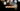# Leetcode | Solution of Unique Email Address in JavaScript

April 3rd, 2020
|

In this post, we will solve unique email addresses problem from leetcode and compute it's time and space complexities. Let's begin.

# Problem Statement

The question can be found at leetcode unique email addresses problem

The problem states that we are given multiple emails in an array and we need to return the number of unique emails in the array based on the following conditions

# Constraints and challenges

The following constraints are given in the question for local names

• The local name of the email can have `.` or `+` in them
• a dot is ignored, so `a.b and ab` are the same
• everything after the first plus is ignored, so `a+bc and a` is same

None of these rules apply to the domain name, so `a.b and ab` are not the same when it comes to domain names. `a+bc and a` are also not the same.

# Solution

The solution includes some string/array processing. Here's the approach

• We'll declare an empty Set to store our processed emails
• Next, we'll iterate over all the emails in the array
• For every email, we'll split the email into local and domain name
• The domain name needs no processing because rules of dot or plus don't apply to them, so we'll divert our focus to local names
• First, we'll ignore everything after the first plus
• Next, we'll replace every dot with an empty string
• This will give us our processed local name
• At last, we'll join the email back and add to set
• Since Set only stores unique values, when the loop exits, the size of the set is the number of unique emails

We have discussed the approach, I urge you to go ahead on leetcode and give it another try.If you are here, it means something went wrong in implementation or you are just too lazy. In any case, let's see a simple implementation of the above logic.
``````var numUniqueEmails = function (emails) {
const set = new Set();

for (const email of emails) {
const arr = email.split("@");

// ignore everything after first occurence of +
arr = (arr.split("+"));

// replace all dots with nothing i.e. empty string
arr = arr.replace(/\./g, "");

// add final email ( processed ) to set
}

return set.size;
};``````

Let's discuss the solution. First, we declare a Set. Next, we loop over all the emails and process them according to the logic discussed above. I guess the comments are self-explanatory in the code snippet about the processing of the local name.

In the end, we return the size of the set, which would only hold unique values.

Here are the stats on submission

``````Status: Accepted
Runtime: 150ms
Memory: 42.3MB``````

## Time and space complexity

### Time complexity

We are looping over the string array once, so time complexity would be O(n).

### Space complexity

We are using extra space to store unique emails. So space complexity is linear, O(n).

# Summary

So, we solved the unique email address problem by simple array and string processing and calculated the time and space complexities.

I hope you enjoyed solving this question. This is it for this one, complete source code for this post can be found on my Github Repo. Will see you in the next one.